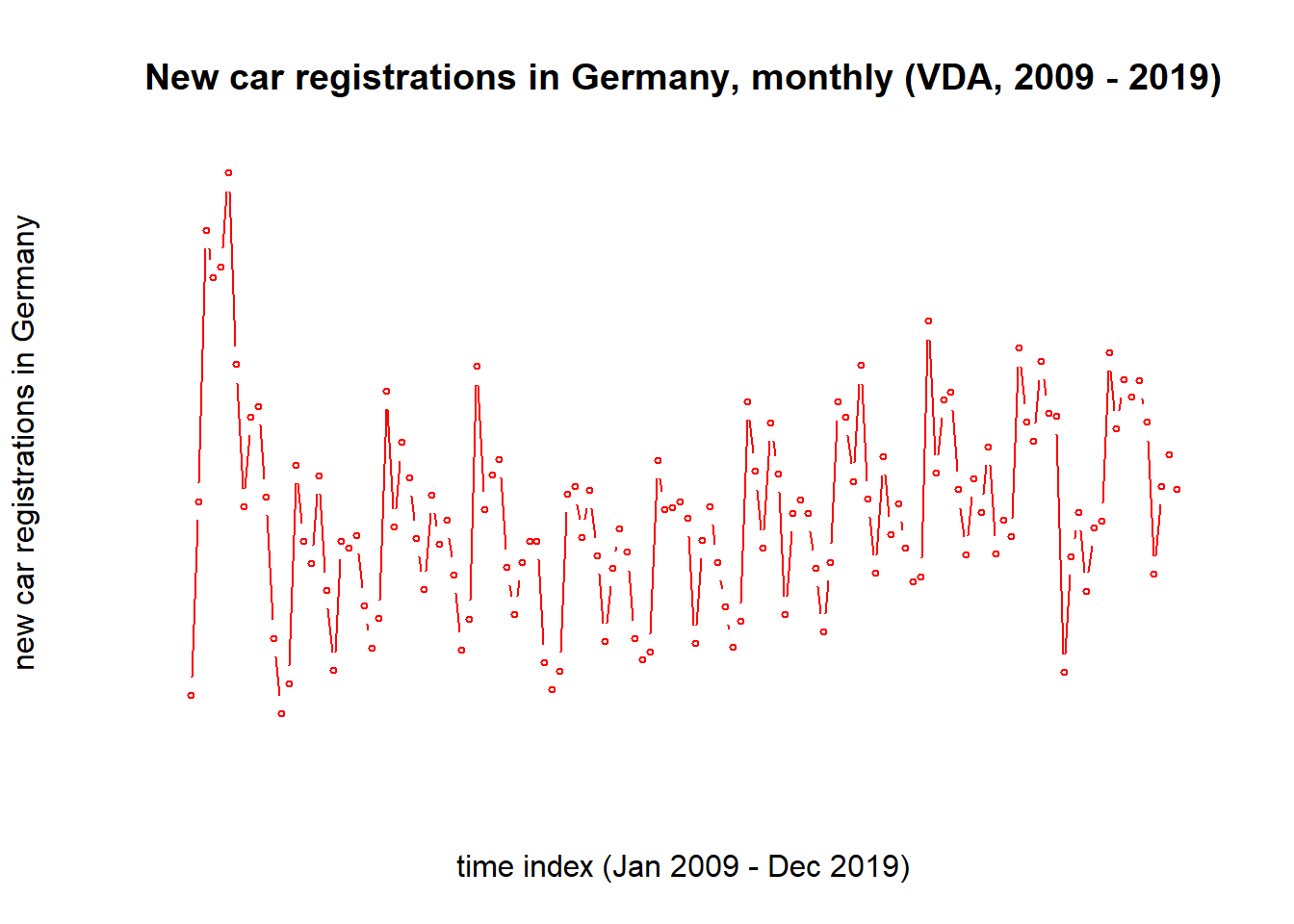# VDA time series data analyzed in RIn previous articles I took a look at US automotive industry. I considered data collected by FRED and US bureau of labor statistics. The data showed strong declines in US automotive production output and US sales of imported vehicles – especially in most recent years. The data also showed that average hourly earnings of employees in US automotive industry have considerably increased in most recent years.

In this article I will take a closer look at German automotive industry by analyzing data provided by VDA. VDA is industry group representing automobile manufacturers in Germany. The data I analyze can be retrieved here: https://www.vda.de/de/services/zahlen-und-daten/monatszahlen.html

The data contains information on monthly production output, export volumes and vehicle registrations in Germany. I exported the data form their website and stored it in a csv-file.

I start my analysis by reading in the data from bespoken csv-file:

``````# use base function to read in csv data
sep=",",
stringsAsFactors = FALSE)

# rename rows
rownames(vda_df) <- c("year",
"month",
"registeredTotal",
"registeredGermans",
"registeredOthers",
"exported",
"produced")

# transpone matrix, using base function "t"
vda_df <- t(vda_df)

# view header of transposed data frame
``````##    year   month     registeredTotal  registeredGermans
## V1 "year" "month"   "Neuzulassungen" "       dt. Marken inkl. Konzernmarken"
## V2 "2009" "Januar"  "189.385"        "128.060"
## V3 "2009" "Februar" "277.740"        "173.452"
## V4 "2009" "MÃ¤rz"    "400.965"        "245.865"
## V5 "2009" "April"   "379.626"        "247.296"
## V6 "2009" "Mai"     "384.578"        "257.070"
##    registeredOthers      exported  produced
## V1 "       ausl. Marken" "Export"  "Produktion"
## V2 "61.325"              "223.281" "310.113"
## V3 "104.288"             "215.549" "297.921"
## V4 "155.100"             "276.910" "435.693"
## V5 "132.330"             "214.966" "373.308"
## V6 "127.508"             "251.119" "427.239"``````

The first row contains the German headers. We do not need them anynmore:

``````vda_df <- vda_df[-1,]
vda_df <- vda_df[1:(11*12),]# I noticed that in my csv-file there were some duplicates, which is why this line was added
``````##    year   month     registeredTotal registeredGermans registeredOthers
## V2 "2009" "Januar"  "189.385"       "128.060"         "61.325"
## V3 "2009" "Februar" "277.740"       "173.452"         "104.288"
## V4 "2009" "MÃ¤rz"    "400.965"       "245.865"         "155.100"
## V5 "2009" "April"   "379.626"       "247.296"         "132.330"
## V6 "2009" "Mai"     "384.578"       "257.070"         "127.508"
## V7 "2009" "Juni"    "427.111"       "290.176"         "136.935"
##    exported  produced
## V2 "223.281" "310.113"
## V3 "215.549" "297.921"
## V4 "276.910" "435.693"
## V5 "214.966" "373.308"
## V6 "251.119" "427.239"
## V7 "312.299" "478.133"``````

Numeric data is currently still considered a character strings. Also, the data is German. The German “.” is the US American “,”. In below code snippet I convert the data into numerics:

``````# take out the "." and convert character strings into numerics
for(i in 3:7){
vda_df[,i] = as.numeric(gsub(pattern=".",
replacement="",
x=vda_df[,i],
fixed=TRUE))
}``````

Now, I can create a time series plot in ggplot2, showing the mont by month absolute figures by relevant category (from Jan 2009 to Dec 2019, according to VDA data). For this I first need to construct a plot-friendly data frame:

``````# create a plot-friendly data frame
values_vc = c()
for(i in 3:7){
values_vc = c(values_vc,vda_df[,i])
}

# create template for plot-friendly data frame
plot_df = as.data.frame(matrix(nrow=132*5,ncol=5))
colnames(plot_df) = c("year","month","timeIndex","series","values")

# populate template (plot_df)
plot_df\$values = values_vc

plot_df\$year = rep(as.vector(vda_df[,1]),
times = 5)

plot_df\$month = rep(as.vector(vda_df[,2]),
times=5)

plot_df\$timeIndex = rep(1:132,
times=5)

plot_df\$series = c(rep("regTotal",times=132),
rep("regGermans",times=132),
rep("regOthers",times=132),
rep("exported",times=132),
rep("produced",times=132))

# show header of populated template
``````##   year   month timeIndex   series values
## 1 2009  Januar         1 regTotal 189385
## 2 2009 Februar         2 regTotal 277740
## 3 2009    MÃ¤rz         3 regTotal 400965
## 4 2009   April         4 regTotal 379626
## 5 2009     Mai         5 regTotal 384578
## 6 2009    Juni         6 regTotal 427111``````
``````# show tail of populated template
tail(plot_df)``````
``````##     year     month timeIndex   series values
## 655 2019      Juli       127 produced 355112
## 656 2019    August       128 produced 311919
## 657 2019 September       129 produced 416400
## 658 2019   Oktober       130 produced 391949
## 659 2019  November       131 produced 417982
## 660 2019  Dezember       132 produced 275920``````

Having produced a plot-friendly data frame I can now visualize the data published by VDA:

``````# load plot and dplyr
library(ggplot2)
library(dplyr)``````
``````##
## Attaching package: 'dplyr'``````
``````## The following objects are masked from 'package:stats':
##
##     filter, lag``````
``````## The following objects are masked from 'package:base':
##
##     intersect, setdiff, setequal, union``````
``````# plot export volume development
x = dplyr::filter(plot_df,series=="exported")
plot(x=x\$timeIndex,
y=x\$values,
type="b",
main = "German automobile export volume, monthly (VDA, 2009 - 2019)",
xlab = "time index (Jan 2009 - Dec 2019)",
ylab = "cars exported from Germany",
col="red",
cex=0.5,
axes = FALSE)``````
``````# plot production output development
x = dplyr::filter(plot_df,series=="produced")
plot(x=x\$timeIndex,
y=x\$values,
type="b",
main = "German automobile production output, monthly (VDA, 2009 - 2019)",
xlab = "time index (Jan 2009 - Dec 2019)",
ylab = "cars produced from Germany",
col="red",
cex=0.5,
axes = FALSE)``````
``````# plot number of vehicles registered in Germany
x = dplyr::filter(plot_df,series=="regTotal")
plot(x=x\$timeIndex,
y=x\$values,
type="b",
main = "New car registrations in Germany, monthly (VDA, 2009 - 2019)",
xlab = "time index (Jan 2009 - Dec 2019)",
ylab = "new car registrations in Germany",
col="red",
cex=0.5,
axes = FALSE)``````

Key take-aways are as follows:

– production output and export levels are back at 2009 levels, which was a year of crisis in automotive industry

– domestic demand is steady and slightly growing

– data suggests correlation between low exports and low production output

The data confirms that German automotive industry is facing difficult times.